## Loop Tests in Underground Cables:

There are several methods for locating the faults in underground cables. However, two popular methods known as Loop Tests in Underground Cables are :

1. Murray loop test
2. Varley loop test

These simple tests can be used to locate the earth fault or short-circuit fault in underground cables provided that a sound cable tuns along the faulty cable. Both these tests employ the principle of Wheatstone bridge for fault location.

### 1.Murray Loop Test:

The Murray loop test is the most common and accurate method of locating earth fault or short-circuit fault in underground cables.

(i) Earth fault: Fig. 11.22 shows the circuit diagram for locating the earth fault by Murray loop test. Here AB is the sound cable and CD is the faulty cable; the earth fault occuring at point F. The far end D of the faulty cable is joined to the far end B of the sound cable through a low resistance link. Two variable resistances P and Q are joined to ends A and C (See Fig. 11.22) respectively and serve as the ratio arms of the Wheatstone bridge.

Let

R = resistance of the conductor loop upto the fault from the test end

X = resistance of the other length of the loop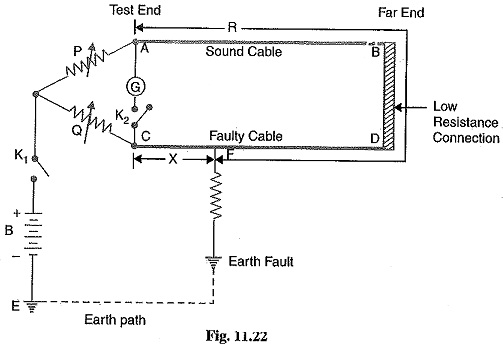Note that P, Q, R and X are the four arms of the Wheatstone bridge. The resistances P and Q are varied till the galvanometer indicates zero deflection.

In the balanced position of the bridge, we have,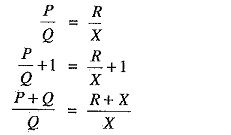If r is the resistance of each cable, then R + X = 2r.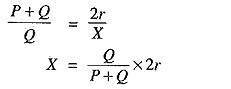If l is the length of each cable in metres, then resistance per metre length of cable = r / l.

Distance of fault point from test end is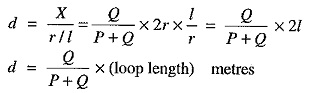Thus the position of the fault is located. Note that resistance of the fault is in the battery circuit and not in the bridge circuit. Therefore, fault resistance does not affect the balancing of the bridge. However, if the fault resistance is high, the sensitivity of the bridge is reduced.

(ii) Short-circuit fault: Fig. 11.23 shows the circuit diagram for locating the short-circuit fault by Murray loop test. Again P, Q, R and X are the four arms of the bridge. Note that fault resistance is in the battery circuit and not in the bridge circuit. The bridge in balanced by adjusting the resistances P and Q. In the balanced position of the bridge :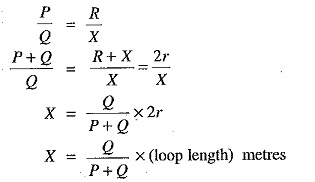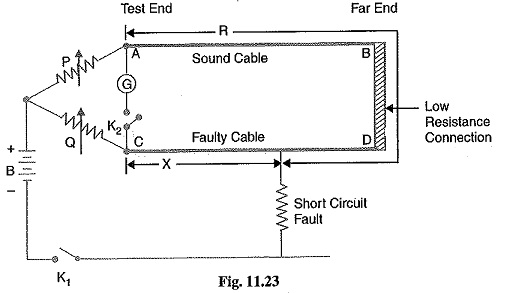Thus the position of the fault is located.

### 2.Varley Loop Test:

The Varley loop test is also used to locate earth fault or short-circuit fault in underground cables. This test also employs Wheatstone bridge principle. It differs from Murray loop test in that here the ratio arms P and Q are fixed resistances. Balance is obtained by adjusting the variable resistance connected to the test end of the faulty cable. The connection diagrams for locating the earth fault and short-circuit fault by Varley loop test are shown in Figs. 11.24 and 11.25 respectively.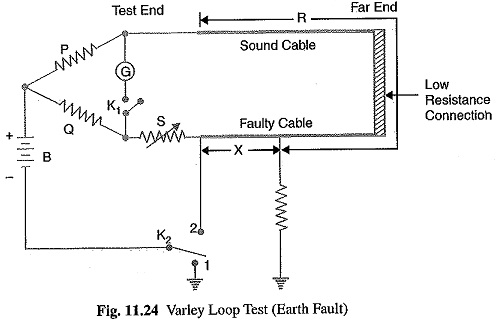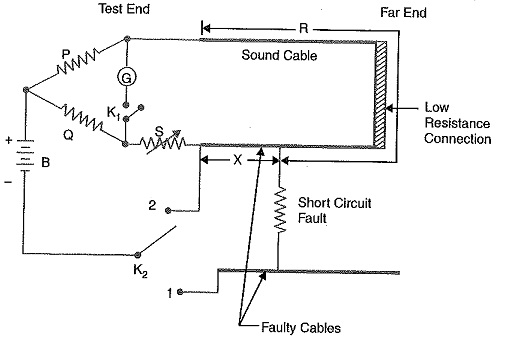For earth fault or short-circuit fault, the key K2 is first thrown to position 1. The variable resistance S is varied till the bridge is balanced for resistance value of S1. Then,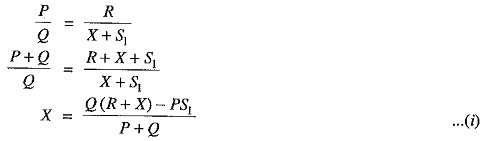Now key K2 is thrown to position 2 (for earth fault or short-circuit fault) and bridge is balanced with new value of resistance S2. Then,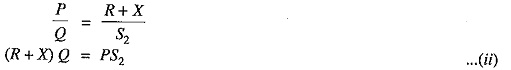From eqs. (i) and (ii), we get,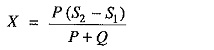Since the values of P, Q, S1 and S2 are known, the value of X can be determined.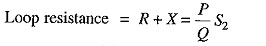If r is the resistance of the cable per metre length, then,

Distance of fault from the Loop Tests in Underground Cables end is

Scroll to Top